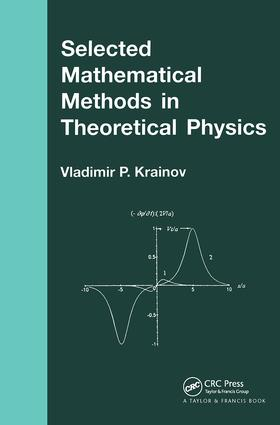Selected Mathematical Methods in Theoretical Physics

1st Edition

CRC Press

210 pages

Purchasing Options:\$ = USD
Paperback: 9780415272391
pub: 2001-10-18
SAVE ~\$17.19
Currently out of stock
\$85.95
\$68.76
x

FREE Standard Shipping!

Description

Selected Mathematical Methods in Theoretical Physics shows how a scientist, knowing the answer to a problem intuitively or through experiment, can develop a mathematical method to prove that answer. The approach adopted by the author first involves the formulation of differential or integral equations for describing the physical procession, the basis of more general physical laws. Then the approximate solution of these equations is worked out, using small dimensionless physical parameters, or using numerical parameters for the objects under consideration. The eleven chapters of the book, which can be read in sequence or studied independently of each other, contain many examples of simple physical models, as well as problems for students to solve. This is a supplementary textbook for advanced university students in theoretical physics. It will enrich the knowledge of students who already have a solid grounding in mathematical analysis.